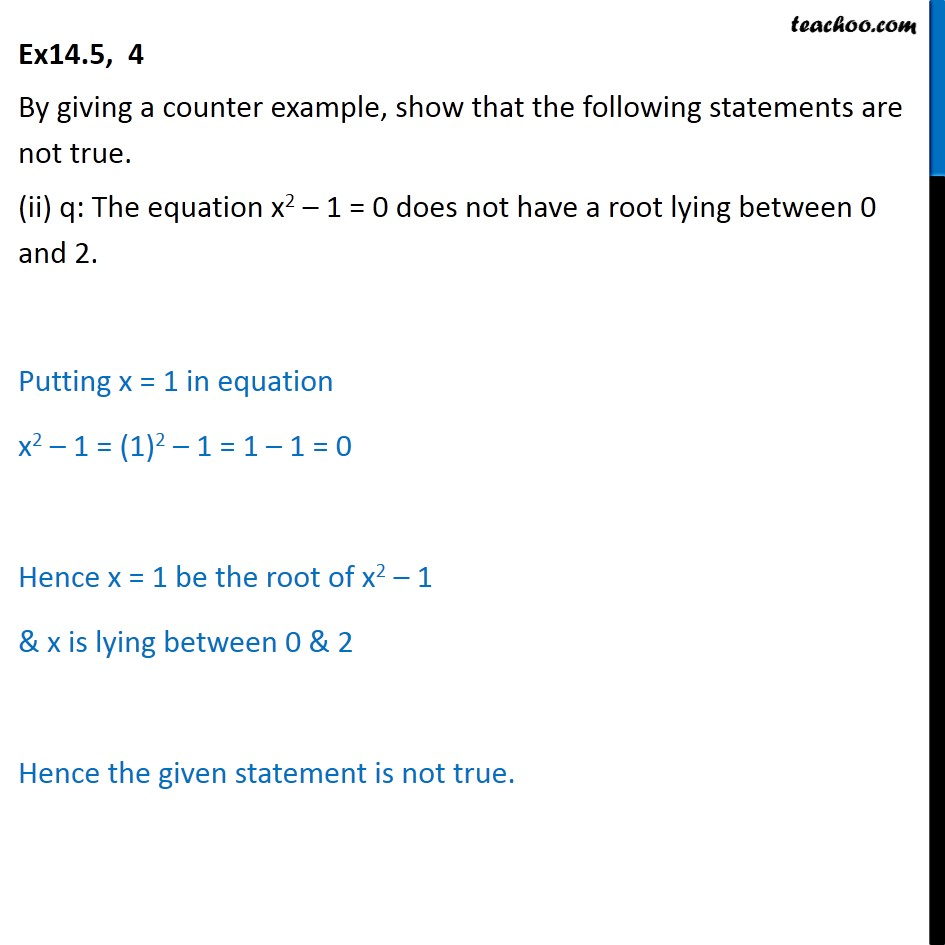Proving Statements true or false

Mathematical Reasoning
Serial order wiseLearn in your speed, with individual attention - Teachoo Maths 1-on-1 Class

### Transcript

Ex14.5, 4 By giving a counter example, show that the following statements are not true. (ii) q: The equation x2 – 1 = 0 does not have a root lying between 0 and 2. Putting x = 1 in equation x2 – 1 = (1)2 – 1 = 1 – 1 = 0 Hence x = 1 be the root of x2 – 1 & x is lying between 0 & 2 Hence the given statement is not true.